# Special problem classes

The following sections describe specializations in Knitro to deal with particular classes of optimization problems. We also provide guidance on how to best set user options and model your problem to get the best performance out of Knitro for particular types of problems.

## Linear programming problems (LPs)

A linear program (LP) is an optimization problem where the objective function and all the constraint functions are linear.

Knitro has built in specializations for efficiently solving LPs. Knitro will automatically detect LPs and apply these specializations, provided the linear structure for the model is added using the API functions for adding linear structure (and not more general callbacks). See Callable library API reference for more detail on API functions for adding linear structure.

A quadratic program (QP) is an optimization problem where the objective function is quadratic and all the constraint functions are linear.

Knitro has built in specializations for efficiently solving QPs. Knitro will automatically detect QPs and apply these specializations, provided the linear and quadratic structure for the model is added using the API functions for adding linear and quadratic structure (and not more general callbacks). See Callable library API reference for more detail on API functions for adding linear and quadratic structure.

Typically, these specializations will only help on convex QPs.

## Systems of nonlinear equations

Knitro is effective at solving systems of nonlinear equations.

There are two ways to try to solve a square system of nonlinear equations using Knitro. In the first way, you can use the least-squares API (see Nonlinear Least-Squares) and just specify the nonlinear equations as the residual functions. Knitro will then formulate your model as an unconstrained optimization problem where the objective function to be minimized is the sum of squares of the nonlinear equations and apply the Gauss-Newton Hessian.

In the second way, you can use the standard Knitro API and specify the nonlinear equations as equality constraints and specify the objective function as zero (i.e., f(x)=0).

The first approach is the recommended approach, however, you should experiment with both formulations to see which one works better.

If Knitro is converging to a stationary point for which the nonlinear equations are not satisfied, the multi-start option may help in finding a solution by trying different starting points.

## Least squares problems

Knitro offers a specialized API for solving least-squares problems,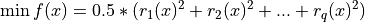with or without bounds on the variables (see Nonlinear Least-Squares). By default, this specialized interface will apply the Gauss-Newton Hessian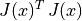where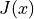is the Jacobian matrix of the residual functions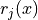at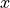. Knitro will behave like a Gauss-Newton method by using the linesearch methods `algorithm` = `KN_ALG_BAR_DIRECT` or `KN_ALG_ACT_SQP`, and will be very similar to the classical Levenberg-Marquardt method when using the trust-region methods `algorithm` = `KN_ALG_BAR_CG` or `KN_ALG_ACT_CG`. The Gauss-Newton and Levenberg-Marquardt approaches consist of using this approximate value for the Hessian and ignoring the remaining term. Using the specialized least-squares interface will generally be the most effective way to solve least-squares models with Knitro, as it only requires first derivatives of the residual functions,, and yet can converge rapidly in most cases.

However, in some cases, if the value of the objective function at the solution is not close to zero (the large residual case), and/or the user can provide the full, exact Hessian matrix, then it may be more efficient to use the standard API and solve the least-squares model as any other optimization problem. Any of the Knitro options can be used.

See knitro_nlnlsq for an implementation in using the Knitro-MATLAB interface.

## Complementarity constraints (MPCCs)

As we have seen in Complementarity constraints, a mathematical program with complementarity (or equilibrium) constraints (also know as an MPCC or MPEC) is an optimization problem which contains a particular type of constraint referred to as a complementarity constraint. A complementarity constraint is a constraint that enforces that two variables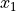and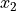are complementary to each other, i.e. that the following conditions hold: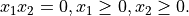These constraints sometimes occur in practice and deserve special handling. See Complementarity constraints for details on how to use complementarity constraints with Knitro.

## Global optimization

Knitro is designed for finding locally optimal solutions of continuous optimization problems. A local solution is a feasible point at which the objective function value at that point is as good or better than at any “nearby” feasible point. A globally optimal solution is one which gives the best (i.e., lowest if minimizing) value of the objective function out of all feasible points. If the problem is convex all locally optimal solutions are also globally optimal solutions. The ability to guarantee convergence to the global solution on large-scale non-convex problems is a nearly impossible task on most problems unless the problem has some special structure or the person modeling the problem has some special knowledge about the geometry of the problem. Even finding local solutions to large-scale, nonlinear, non-convex problems is quite challenging.

Although Knitro is unable to guarantee convergence to global solutions it does provide a multi-start heuristic that attempts to find multiple local solutions in the hopes of locating the global solution. See Multi-Start.

## Mixed integer programming (MIP)

Knitro provides tools for solving optimization models (both linear and nonlinear) with binary or integer variables. See the dedicated chapter Mixed-integer nonlinear programming for a discussion on this topic.